# Proportion

Here we will learn about proportion in maths, including direct and inverse proportion, real life examples, worded problems, and graphical representations.

There are also proportion in maths worksheets based on Edexcel, AQA and OCR exam questions, along with further guidance on where to go next if you’re still stuck.

## What is proportion?

Proportion is a type of relationship between two variables linked by a constant.

There are two types of proportion, direct proportion and inverse proportion. They can also be referred to as direct variation and inverse variation.

When solving problems involving proportion it is important to know which type of proportion that you are dealing with, direct proportion or inverse proportion.

Step-by-step guide: Direct and indirect proportion

• Direct proportion

If there is a directly proportional relationship between two variables then as one variable increases, so does the other.

For example,
An apple costs 60p. As the number of apples increases, so does the cost.

The relationship between the number of apples and the cost of the apples is directly proportional.

\text{Cost} = \text{number of apples} \times 60

The constant in this example is 60.

Step-by-step guide: Direct proportion

• Inverse proportion

If there is an inversely proportional relationship between two variables then as one variable increases, the other variable decreases.

For example,
1 worker takes 30 days to paint a long fence. As the number of workers increases, the time it takes to paint the fence decreases.

The relationship between the number of workers and the time taken to paint the fence is inversely proportional.

\text{Days to paint the fence} = \frac{30}{\text{number of workers}}

The constant in this example is 30.

Step-by-step guide: Inverse proportion

### What is proportion?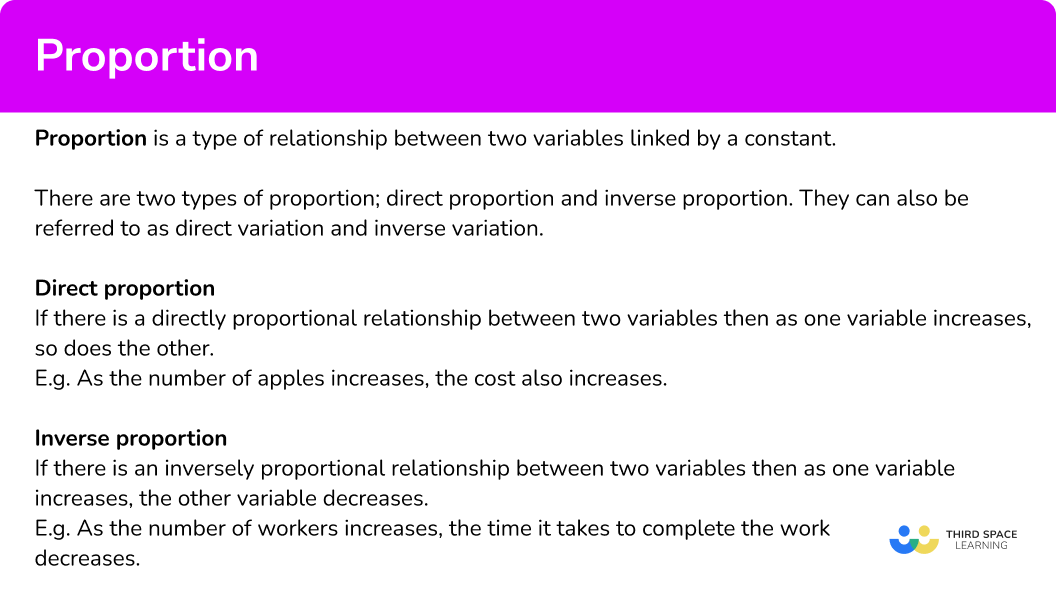### Solve ratio and proportion problems

Sometimes a proportion problem may also involve ratio.

For example,

A cafe sells apples and bananas in the ratio 2:3. They sell 35 pieces of fruit altogether. Apples cost 30p and bananas cost 45p. Work out the total amount of money the fruit cost.

So, first you use the ratio to work out how many apples and bananas were sold. The ratio 2:3 has 5 parts, so you divide 35 by 5 to get 7. Then you multiply each part of the ratio by 7. This means that 14 apples and 21 bananas were sold.

\begin{aligned} A&: B \\\\ 2 &: 3\\\\ 2\times 7&:3\times 7\\\\ 14&:21 \end{aligned}

You can then use direct proportion to find the price of the apples and the price of the bananas.

Apples: 14\times 30=420

Bananas: 21\times 45=945

We add these together to get the total price for all the fruit.

420+945=1365

Therefore the total price is 1365p or £13.65.

### Notation for proportional relationships

This symbol ‘\bf{\propto}’ means ‘is proportional to’.

If y is directly proportional to x you can write it as y \propto x.

If y is inversely proportional to x you can write it as y \propto \frac{1}{x}.

### Proportion formulas and the constant of proportionality

Proportion is a type of relationship between two variables linked by a constant. This is known as the constant of proportionality and is usually denoted by the letter ‘k’.

Equations of proportion:

• The direct proportion formula is, y=kx .

Step-by-step guide: Direct proportion formula

• The inverse proportion formula is, y=\frac{k}{x}.

Step-by-step guide: Inverse proportion formula

### Graphs of proportional relationships

Proportional relationships can be represented graphically.

For direct proportion, as one variable increases the other variable increases.

Therefore, the graph will go up as you look from left to right. The graph can be a straight line or a curve. All direct proportion graphs must start at the origin.

For example,

If y is directly proportional to x then the graph will be a straight line.

If y is directly proportional to a function of x such as x^{2} or \sqrt{x} then the graph will form a curve.

For inverse proportion, as one variable increases the other variable decreases.

Therefore, the graph will go down as you look from left to right. All inverse proportion graphs form a curved shape like in the example below.

Whether y is inversely proportional to just x or a function of x it will still form a curved graph with this general shape.

Step-by-step guide: Directly proportional graph / inversely proportional graph

### Types of proportion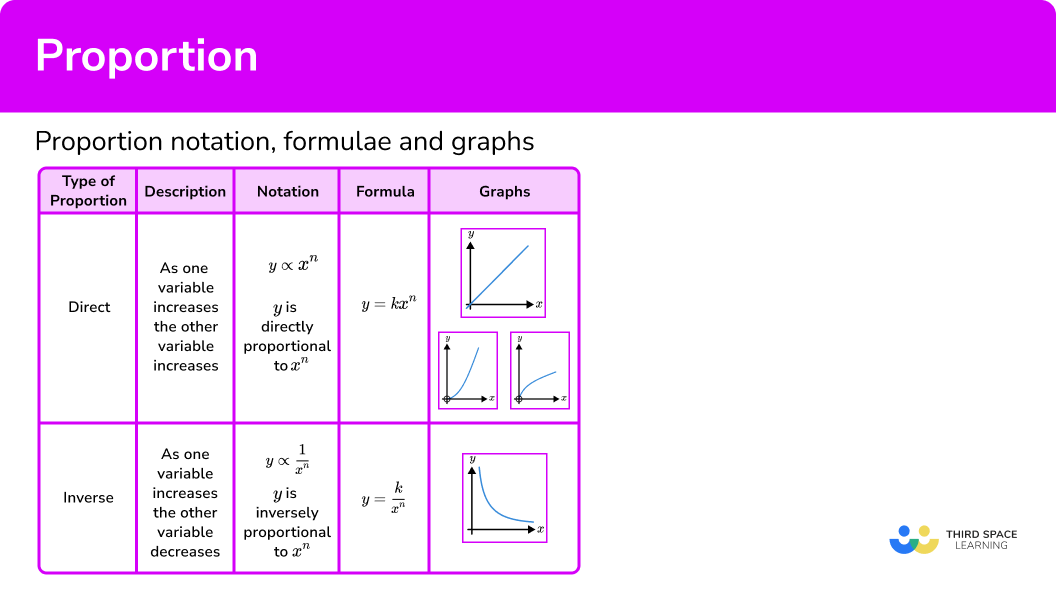## How to use proportion in maths

In order to answer general proportion in maths problems:

1. Identify if the relationship between the variables in the question is
(a) directly proportional.
(b) inversely proportional.
2. (a) By using division, find the constant.
(b) By using multiplication, find the constant.
3. (a) Multiply the constant by the required value of one variable to find the answer for the other variable.
(b) Divide the constant by the required value of one variable to find the answer for the other variable.

### Explain how to use proportion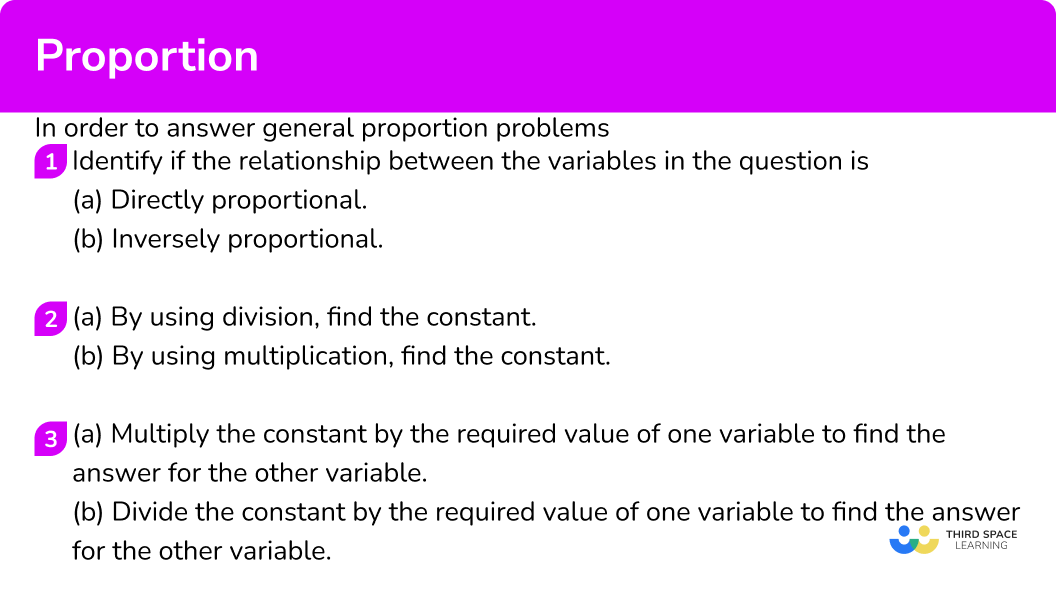## Proportion examples

### Example 1: worded problem

If 5 biscuits cost 80p, find the cost of 7 biscuits.

1. Identify if the relationship between the variables in the question is
(a) directly proportional.
(b) inversely proportional.

As the number of biscuits increases the cost will also increase. Therefore the variables are directly proportional.

2(a) By using division, find the constant.
(b) By using multiplication, find the constant.

80 \div 5 = 16

1 biscuit will cost 16p.

3(a)  Multiply the constant by the required value of one variable to find the answer for the other variable.
(b)  Divide the constant by the required value of one variable to find the answer for the other variable.

16 \times 7 = 112

7 biscuits will cost 112p or £1.12.

You can also visualise these steps in a table.

### Example 2: worded problem

If 6 bags of flour weigh 15 \ kg, find the weight of 17 bags of flour.

As the number of bags of flour increases the weight will also increase. Therefore the variables are directly proportional.

15 \div 6 = 2.5

1 bag of flour will weigh 2.5kg.

2.5 \times 17 = 42.5

17 bags of flour will weigh 42.5kg.

You can also visualise these steps in a table.

### Example 3: worded problem

If 4 taps pumping water at a constant rate take 9 hours to fill a swimming pool, find the time it would take 3 taps pumping water at the same rate to fill the swimming pool.

As the number of taps increases the time it takes to fill the swimming pool decreases. Therefore the variables are inversely proportional.

4 \times 9 = 36

It would take 1 tap 36 hours to fill the pool.

36 \div 3 = 12

3 taps would take 12 hours to fill the pool.

You can also visualise these steps in a table.

### Example 4: worded problem

A local park has a course marked out for runners to use. One runner completes the course in 30 minutes running at a speed of 8 \ km/h.

Another runner has a pace of 12km/h. How long will it take this runner to complete the course?

As the speed increases, the time taken to complete the course decreases. Therefore the variables are inversely proportional.

30 \times 8 = 240

The constant for the course is 240.

If your speed was 1km/hour it would take 240 minutes.

240 \div 12 = 20

It would take the runner 20 minutes to complete the course.

You can also visualise these steps in a table.

Note that this question can also be answered using the formula for speed.

Step-by-step guide: Speed distance time

## Calculations with proportions

In order to answer problems involving a proportion formula:

1. Identify the type of proportion and write down the appropriate formula.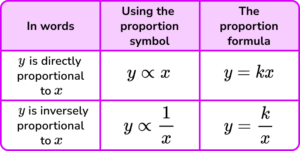2. Substitute in the given values and rearrange the resulting equation to determine the value of \textbf{k} .
3. Substitute the value of \textbf{k} into the formula written down in step 1.

### Example 5: writing a proportion formula

y is directly proportional to x.

When y=12, \ x=3.

Find a formula for y in terms of x.

y=kx

\begin{aligned} y&= k \times x\\\\ 12 &= k \times 3\\\\ 12 \div 3 &= k\\\\ 4&=k \end{aligned}

\begin{aligned} &y=kx\\\\ &k=4 \\\\ &y=4x \end{aligned}

### Example 6: writing a proportion formula

a \propto \frac{1}{b}

When a=6, \ b = 5.

Find a formula for a in terms of b.

a = \frac{k}{b}

\begin{aligned} a &= \frac{k}{b} \\\\ 6 &= \frac{k}{5} \\\\ 6 \times 5 &= k \\\\ 30&=k \end{aligned}

\begin{aligned} &a = \frac{k}{b}\\\\ &k=30 \\\\ &a = \frac{30}{b} \end{aligned}

### Common misconceptions

• Assuming worded problems reflect real life

Worded proportion problems can often imply things that are not reflective of real life.

For example,

It takes 3 workers 2 days to build a wall, how long does it take 4 workers to build the same wall? This question makes an assumption that all workers work the same hours each day and are equally efficient. This is not reflective of real life, but it is something we assume in order to model the situation mathematically.

• Assuming the unitary method is the only/best way to solve a proportion problem

There may be several ways to get to the correct answer for proportion questions. Some ways are more efficient than others depending on the numbers involved. You can always follow the step by step guide, however it is good practice to think independently about each question and consider other methods to develop your problem solving skills.

If the solution to a proportion problem is a decimal, think carefully about what this represents in the context of the question before writing your final answer.

For example,

5.3 pounds should be written as £5.30.

2.5 hours could be written as 2 hours 30 minutes.

### Practice proportion questions

1. If 8 batteries cost £4.80, find the cost of 5 batteries.

£3.00£12.00£0.60£7.68This is a direct proportion problem.

The cost of one battery would be,

4.80\div 8=0.6.

The cost of 5 batteries would be,

0.6\times 5=3.

2. If 3 paving slabs weigh 18 \ kg, find the weight of 7 slabs.

7.7 \ kg378 \ kg42 \ kg6 \ kgThis is a direct proportion problem.

The weight of one slab would be,

18\div 3=6.

The weight of 7 slabs would be,

6\times 7=42.

3. If a taxi journey is shared by 4 people it costs £24 each. What does each person pay if there are only 3 people sharing the taxi for the same journey?

£18£32£20£24This is an inverse proportion problem.

The cost of the whole taxi journey would be,

4\times 24=96 pounds.

Sharing this cost between 3 people would be,

96\div 3=32.

4. If it takes 5 workers 12 hours to do a job, how long would it take 9 workers to complete the same job? Give your answer in hours and minutes.

3 hours 45 minutes21 hours 6 minutes6 hours 66 minutes6 hours 40 minutesThis is an inverse proportion problem.

The total time needed to complete the job would be,

5\times 12=60 hours.

Sharing this time between 9 workers would be,

60\div 9=6\frac{2}{3}.

Two thirds of an hour is 40 minutes,
60 \times \frac{2}{3} = 40 .

The final answer would be 6 hours 40 minutes.

5. Which of these equations indicates that y is directly proportional to x?

y=5xy=x+5y=\frac{5}{x}y=5-xThe correct equation will be in the form y=kx, where k is the constant of proportionality.

The correct equation will be, y=5x.

6. Which of these graphs indicates that y \propto x?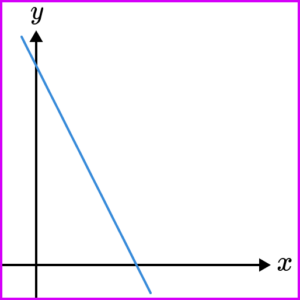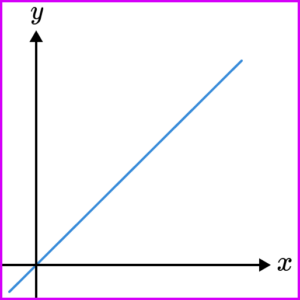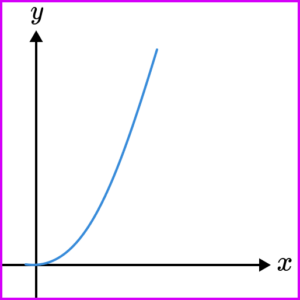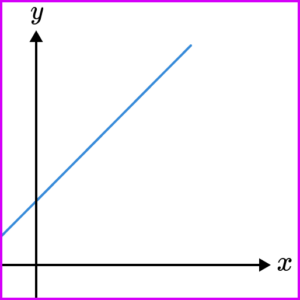y \propto x means y is proportional to x.
Therefore the graph should show that as x increases, y increases.

All direct proportion graphs go through the origin (0,0).
As y is proportional to x (and not x^{2} \text{or} \sqrt{x} for example) the graph will be a straight line.

7. y is inversely proportional to x. When y=20, \ x=4. Find a formula for y in terms of x.

y=\frac{80}{x}y=5xy=\frac{5}{x}y=80xNow substitute in the values of x and y and rearrange the resulting equation to find k.

\begin{aligned} 20 &= \frac{k}{4} \\\\ 20 \times 4 &= k \\\\ 80&=k \end{aligned}
Finally, substitute this value of k into the general formula to get the final answer

y = \frac{80}{x}.

### Proportion GCSE questions

1. A recipe to make enough garlic bread for 5 people needs 75 \ g of butter.

Calculate the amount of butter needed to make garlic bread for 12 people.

(2 marks)

75 \div 5 = 15

(1)

15 \times 12 = 180g

(1)

2. y is inversely proportional to x.

Complete the table.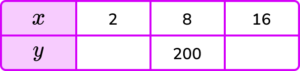(2 marks)

8 \times 200 = 1600 1600 \div 16 = 100

(1)

1600 \div 2 = 800

(1)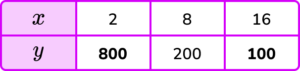3. The graphs show different relationships between the variables x and y.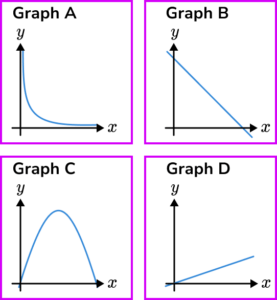(a) Identify the graph which shows that y\propto x .

(b) Identify the graph which shows that y\propto \frac{1}{x} .

(2 marks)

(a) Graph D

(1)

(b) Graph A

(1)

## Learning checklist

You have now learned how to:

• Solve real world problems involving direct and inverse proportion
• Recognise formula and graphs for direct and inverse proportions

## Still stuck?

Prepare your KS4 students for maths GCSEs success with Third Space Learning. Weekly online one to one GCSE maths revision lessons delivered by expert maths tutors.

Find out more about our GCSE maths tuition programme.